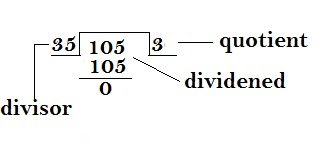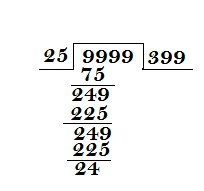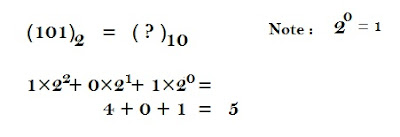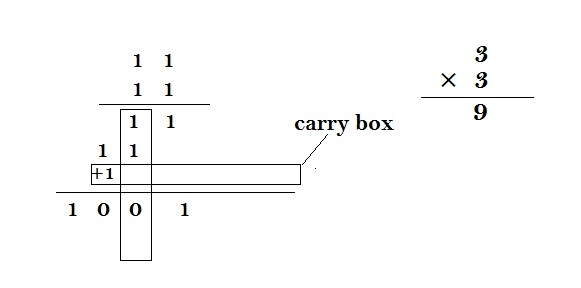New Students Offer - Use Code HELLO

# Number System Guide

Complete guide to number system :-• Number and Classification
• Divisibility and Property
• Remainder and it's Rules
• Sum
• Last Digit
• Number of Zeroes at the end of the product
• Binary Number

### Number and Classification

Number system is one of the most important topic for understanding basics of mathematics and quantitative ability. I have noted down some short notes on number system for the competitive exams.
• Natural Number:- The most familiar numbers are the natural numbers :Example 1, 2, 3, and so on.(except 0)
• Whole Number:- Natural Number + Zero. Example 0,1,2….. etc
• Integer:- a number that can be written without a fractional component. Example -1,-2,-3…, 0…,1,2,3 are integers,
• Rational number:- a number that can be expressed as a fraction . Example 1/7, 8/15 etc.
• Real number:- It include all the rational numbers, such as the integer −5 and the fraction 4/3, and all the irrational numbers. √2 (1.41421356... the square root of 2
• Imaginary Numbers: Imaginary numbers are those numbers which we can just imagine but cannot physically perceive.
• For Example √-1, √-3 etc, √-1 is represented by i, Square Root of all imagenary number is imaginary.
• Complex Number:- Combination of Real and Imaginary number is called complex numbers. Example: (2+5i) , (1+3i) etc.
• Even number:- an integer that is "evenly divisible" by two. Example 2,4,8,….. etc.
• Odd number:- all the Natural numbers which are not Even numbers. Example 1, 3, 19, 21,….. etc.
• Prime number:- an integer greater than 1 which are only divisible by 1 and the number itself are called prime numbers. Example 2,3,5,7,11,13,….etc
• Co-Prime Number:- if the only positive integer (factor) that divides both of them is 1. Examples:- 13 and 14 are co-prime, being commonly divisible only by 1, while 22 and 24 are not co-prime, because they are both divisible by 2.
• Composite Number:- All the natural numbers greater than 1 which are divisible by at least one more number other than 1 and the number itself are called composite numbers. Example: 6 can be divided exactly by 3 or 2 (as well as 1 and 6), so 6 is a composite number. But 7 cannot be divided exactly (except by 1 and 7), so is NOT a composite number (it is a prime number).
• Place value:- The place or position of a digit in a number is called its place value. Example: Place value of 2 in 4289 is 200.
• Face value:- The real value of any digit in a certain number is called its face value. Example: face value of 2 in 7246 is 2.
Some short notes on number system for the exam point of view.
1. Number 1 is neither divisible nor prime.
2. Two consecutive odd prime numbers are called prime pair.
3. All natural numbers are whole, rational, integer and real.
4. All whole numbers are rational Integer and real.
5. All whole numbers are rational and real.
6. All rational numbers includes the integers, since every integer can be written as a fraction with denominator 1. Example (7/1) not only 7
7. All whole numbers, rational and irrational numbers are real.
8. Natural (including Prime, Composite, even or odd) numbers and whole numbers are never negative.
9. Any Prime Number can never be a Composite Number.
10. Fractions are rational.
11. All prime number except 2 are odd. Or 2 is the only even prime number.
12. Zero (0) is neither negative nor positive number.
13. If a is any number then, if a divides zero, result will be zero. If 0 divides a, then result will be infinite or not defined or undetermined i.e.
• 0/a =0, but a/0 =∞ (infinite)
• where a is real number.
14. The sum and the product of two rational number is always a rational numbers.
15. The product or the sum of a rational number and irrational number is always an irrational number.
16. Pie (π) is an irrational number.
17. There can be infinite number of rational or irrational numbers between two rational numbers or two irrational numbers.
18. Decimal indication of an irrational number is infinite coming.as−√3,√2as−3,2
19. The square of an even is even and the square of an odd number is odd. DG

## Question-based on Number system

### Question.1:- Zero is what type of number?

1) Whole number
2) Prime number
3) Real number
4) Both (1) and (2)
5) Both (1) and (3)
Explanation:- option (5), Whole number = 0,1,2,3…
Real number = …….-3, -2, -1, 0, 1, 2, 3…. And …… 2.3,-4.8…..
Prime number not contain zero

### Question.2:- -4 is what type of number?

1) Real number
2) Integer
3) Whole number
4) Both (1) and (2)
5) None of these
Explanation:- option (4), Real number = …….-3, -2, -1, 0, 1, 2, 3…. And ……. 2.3,-4.8…..
Integer = …….-3, -2, -1, 0, 1, 2, 3….
Whole number not contain Negative number

### Question.3:- Which of the following are Whole number?

1) 4
2) -4
3) ¼
4) 0.25
5) None of these
Explanation:- option (1), Whole number = 0,1,2,3,4…..

### Question.4:- Which one is True?

a) All Whole number can be Natural number But not Vice versa
b) All Natural number can be Whole number But not Vice versa
c) Zero is a prime number
d) 4 is a Composite number
1) (a) and (c)
2) (a) and (d)
3) (b) and (d)
4) (b) and (c)
5) (c) and (d)
Explanation:- option (3), Whole number = Natural number + Zero
Prime number = 1, 3, 5, 7, 11…… (not contain Zero)
Composite number = 4 can be divided by (1,2,4), so it can be.

### Question.5:- What is the second smallest Odd Prime number?

1) 1
2) 5
3) 3
4) 2
5) None of these
Explanation:- option (2), Prime number (2,3,5….) are odd number except 2.

### Question.6:- Which of the following are Co-Prime Number?

1) (4,5)
2) (4,8)
3) (9,6)
4) (12,9)
5) None of these
Explanation:- option (1), only positive integer (factor) that divides both of them is 1.

### Question.7:- Which number is neither Prime nor Composite?

1) 15
2) 41
3) 18
4) 1
5) None of these
Explanation:- option (4), 15 and 18 are Composite numbers
41 is a Prime number

### Question.8:- All Prime number are Odd. Is it?

a) True
b) False
c) True except 2
d) False except 1
1) (a) and (c)
2) (b) and (c)
3) (a) and (d)
4) (b) and (d)
5) (c) and (d)
Explanation:- option (2), Prime number = 2,3,5,7,11,13……. Odd except 2

### Question.9:- What is the Place value of 9 in 150592 ?

1) 90
2) 900
3) 92
4) 09
5) None of these
Explanation:- option (1), Position of 9 in 150592 is 90

### Question.10:- Which number is Rational but not Integer?

1) 3
2) 0
3) 4
4) 2.5
5) Pie (π)
Explanation:- option (4), 0,4,3 are Integers and Pie (π) is an irrational number

### Divisibility and Property

 Numbers Divisibility Test 2 All even number(including 0) is divisible by 2 3 If the sum of digit of a number is divisible by 3 then the number is also divisible by 3. 4 If the last two digit of the number is divisible by 4 then the number is also divisible by 4(like 400). 5 If the number ends with 5 or 0. 6 If the number exactly divides by 2 and 3 then it also divisible by 6. 8 If the last three digit of the number is divisible by 8 then the number is also divisible by 8.(like 4ooo) 9 If The sum of the digit of number divisible by 9 then the number is also divisible by 9.

Properties of Divisibility
• If a number A is divisible by B then the factors of A is also divisible by B.
• If a number A divides p then the sum of digits of p is also divisible by B.
• If a number A divides p then the difference of digits of p is also divisible by B.

### Remainder and its rule

If a number  A divide by B then what would happen. Either it leaves remainder or not(means completely divides).

Case 1:(leaves remainder)dividend = divisor × quotient + remainder
A = B × Q + R
Case 2. (leaves no remainder )dividend = divisor × quotient
A = BQ

Making a number completely or exactly divisible to given number
There is a two way if we want that the given number should not leaves any remainder and get exactly divides with the divisor or any number.we have two method

Method 1: Subtracting the remainder with the given number or dividend . It is also used in finding the greatest n digit no exactly divisible by the given number.

Method 2: Subtract the divisor from remainder and then add with dividend.It is also used in finding the lowest n digit no exactly divisible by the given number.
Example:Not to make 1326 exactly divisible with 12 we have two method by following:

Method (1):
1326-6 = 1320

Method (2):
1326+12-6 = 1332

Finding Greatest n digit number exactly divisible by the given number
Find the greatest 4 digit number exactly divisible by 25.
Sol:Applying Method (1) :
9999-24 = 9975

Find the least 4 digit number exactly divisible by 28
Sol:
1000 + 28 - 20 = 1008

### Sum

Find the sum of all natural number from 1 to 50 ?
Sol:
1+2+3+4+..........+50 = n(n+1)/2
50×51/2 =  2550/2 = 1275

Find the sum of even numbers from 2 to 10 ?
Sol:
2+4+6+8+10 = n(n+1)
5×6 = 30

Find the sum of odd number from 1 to 17 ?
Sol:
1+3+5+7+9+11+13+15+17 = (n)2
(9)2 = 81

Find the sum of squares from 1 to 18 ?
Sol:
(1)2+(2)2+(3)2+(4)2.....+(18)= n(n+1)(2n+1)/6
18×19×37/6 = 12654/6 = 2109

Find the sum of cubes from 1 to 12 ?
Sol:
(1)3+(2)3+(3)3+(4)3.....+(12)= [n(n+1)/2]2
[12×13/2]2 = 156/2 = (78)2 = 5084

### Last Digit

Following Table will help you in finding a last digit for any number.Just Let the last digit of the number as a Z.Find the last digit of (20373)130 ?
Sol:
(20373)n , here n = 130 and Z = 3
130 divide by 4 leaves remainder = 2
the last digit for (20373)130 = Z2
which means 3= 9.

Find the last digit (5548)228 ?
Sol:
here n = 228, n divide by 4.
228/4 leaves remainder = 4
last digit (8)4 = 4096
which means 6

### Number of Zeroes at the end of the Product

To find the number of zeroes at the end of the product we need to know the number of squares of 2 and 5 in a multiplication.

#### Example :

Find the Number of zeroes at the end of :
45×15×42×32×14×22 ?
Sol:
(5×9) + (5×3) + (21×2) +(2)+ 2×7 + 2×11
No. of 2's(only 2) = 21+5+1+1
no. of 2's = 8
No. of 5's (only 5) = 51+1
no. of 5's = 2
So pick whichever has contains the least number of digit from 2 and 5 only
no. of 2's = 8
no. of 5's = 2
because here in this case is 2 hence number of zeroes at the end is two.

### Binary Number

The number which we knew is 0,1,2,3,4,5,6,7,8,9. this number system is known as decimal number which contains 10 digits from zero to ten therefor it has base 10. If we already have a number system which is  decimal number system so why we need binary number because computer only understand either 1 or 0.

As in decimal number we perform mathematical operation like subtraction , addition and multiplication same happens in case of binary number.

First of all we understand how to convert a decimal number into binary number and binary number into decimal number.

Binary number has its base 2 because it has only two digits 0 and 1. and the decimal number has its base 10 because it has only ten digits from 0 to 9.

Conversion of decimal number into binary number
Convert (212)10 into ( ? )2 ?
Sol:Convert (101)2 into ( ? ) = ?
Sol:some mathematical operation
• Subtraction
• Multiplication

Rule :
0 + 0 = 0
0 + 1 = 1
1 + 0 = 1
1 + 1 = 0 carry = 1#### Subtraction

1 - 0 = 1
1 - 1 = 0
0 - 0 = 0
0 - 1 = 1 carry = 1#### Multiplication

0 × 0 = 0
0 × 1 = 0
1 × 0 = 0
1 × 1 = 1
Example :